﻿ Audio Compression Using DWT and RLE TechniquesPublications are Open
Access in this journal
Article Versions
Export Article
• Normal Style
• MLA Style
• APA Style
• Chicago Style
Research Article
Open Access Peer-reviewed

### Audio Compression Using DWT and RLE Techniques

Abebe Tsegaye , Girma Tariku
American Journal of Electrical and Electronic Engineering. 2019, 7(1), 14-17. DOI: 10.12691/ajeee-7-1-3
Received March 17, 2019; Revised April 26, 2019; Accepted May 13, 2019

### Abstract

The growth of the cellular technology and wireless networks all over the world has increased the demand for digital information by manifold. This massive demand poses difficulties in handling huge amounts of data that need to be stored and transferred. To overcome this problem we can compress the information by removing the redundancies present in it. Redundancies are the major source of generating errors and noisy signals. Coding in MATLAB helps in analyzing compression of audio signals with varying bit rate and remove errors and noisy signals from the audio signals. The audio signal’s bit rate can also be reduced to remove errors and noisy signals which are suitable for remote broadcast lines, studio links, satellite transmission of high quality audio and voice over internet. This paper focuses on the audio compression process and its analysis using DWT and RLE Techniques through MATLAB by which processed audio signal can be heard with clarity and in the noiseless mode at the receiver end.

### 1. Introduction

The growth of the computer industry has invariably led to the demand for quality audio data. Compared to most digital data types, the data rates associated with uncompressed digital audio are substantial. For example, if we want to send high-quality uncompressed audio data over a modem, it would be gradually received, stored away and the resulting file played at the correct rate to hear the sound. However, if real time audio is to be sent over a modem link, data compression must be used. 2

The idea of audio compression is to encode audio data to take up less storage space and less bandwidth for transmission. To meet this goal different methods for compression have been designed. Just like every other digital data compression, it is possible to classify them into two categories: lossless compression and lossy compression. 1

DWT is a highly flexible family of signal representations that may be matched to a given signal and it is well applicable to the task of audio data compression. In this case the audio signal will be divided into overlapping frames of length 2048 samples (46 ms at 44.1 kHz). The two ends of each frame are weighted by the square root of a Hanning window of size 128 to avoid border distortions. 3

A technique to incorporate psychoacoustic models into an adaptive wavelet packet scheme to achieve perceptually transparent compression of high-quality (44.1 kHz) audio signals at about 45kb/s. The filter bank structure adapts according to psychoacoustic criteria and according to the computational complexity that is available at the decoder. This permits software implementations that can perform according to the computational power available in order to achieve real time coding/decoding. 5

1.1. Introducing Wavelets

The fundamental idea behind wavelets is to analyze according to scale. The wavelet analysis procedure is to adopt a wavelet prototype function called an analyzing wavelet or mother wavelet. Any signal can then be represented by translating and scaled versions of the mother wavelet. Wavelet analysis is capable of revealing aspects of data that other signal analysis techniques such as Fourier analysis miss aspects like trends, breakdown points, discontinuities in higher derivatives, and self-similarity. Furthermore, because it affords a different view of data than those presented by traditional techniques, it can compress or de-noise a signal without appreciable degradation. It has two parts in wavelet scaling and shifting. 7

1.2. Scaling

Simply put Scaling a wavelet means stretching (or compressing) it. To go beyond colloquial descriptions such as "stretching," we introduce the scale factor, often denoted by the letter ‘a’. If we're talking about sinusoid, The scale factor works exactly the same with wavelets. The smaller the scale factor, the more "compressed" the wavelet and vice versa.

1.3. Shifting

Shifting a wavelet simply means delaying (or hastening) its onset. Mathematically, delaying a function f (t) by ‘b’ is represented by f (t-b).

### 2. System Analysis and Design

2.1. Wavelet and Audio Compression

The idea behind signal compression using wavelets is primarily linked to the relative scarceness of the wavelet domain representation of the signal. Wavelets concentrate speech information (energy and perception) into a few neighboring coefficients. Therefore, as a result of taking the wavelet transform of a signal, many coefficients will either be zero or have negligible magnitudes. 9

Another factor that comes into picture is taken from psychoacoustic studies. Since our ears are more sensitive to low frequencies than high frequencies and our hearing threshold is very high in the high frequency regions, we used a method for compression by means of which the detail coefficients (corresponding to high frequency components) of the wavelet transform are threshold such that the error due to threading is inaudible to our ears.

In summary, the notion behind compression is based on the concept that the regular signal component can be accurately approximated using the following elements: a small number of approximation coefficients (at a suitably chosen level) and some of the detail coefficients.

Data compression is then achieved by treating small valued coefficients as insignificant data and thus discarding them. The process of compressing an audio signal using wavelets involves a number of different stages, each of which is discussed below.

2.2. Audio Signal

The sample speech files used for compression are .wav files. These files contain discrete signal values, which can be easily read and played by MATLAB at a sampling frequency of 8 KHz.

2.3. Choice of Wavelet

The choice of the mother-wavelet function used in designing high quality speech coders is of prime importance. Several different criteria can be used in selecting an optimal wavelet function. The objective is to minimize reconstructed error variance and maximize signal to noise ratio (SNR). In general optimum wavelets can be selected based on the energy conservation properties in the approximation part of the wavelet coefficients.

2.4. Wavelet Decomposition

Wavelets work by decomposing a signal into different resolutions or frequency bands, and this task is carried out by choosing the wavelet function and computing the Discrete Wavelet Transform (DWT). Signal compression is based on the concept that selecting a small number of approximation coefficients (at a suitably chosen level) and some of the detail coefficients can accurately represent regular signal components 10.

2.5. Truncation of Coefficients

After calculating the wavelet transform of the audio signal, compression involves truncating wavelet coefficients below a threshold. From the experiments that we conducted, we found that most of the coefficients have small magnitudes. The audio in general terms, more than 90% of the wavelet coefficients were found to be insignificant, and their truncation to zero made an imperceptible difference to the signal. This means that most of the audio energy is in the high-valued coefficients, which are few. Thus the small valued coefficients can be truncated or zeroed and then be used to reconstruct the signal.

2.6. Encoding

Signal compression is achieved by first truncating small-valued coefficients and then efficiently encoding them. One way of representing the high-magnitude coefficients is to store the coefficients along with their respective positions in the wavelet transform vector. Another approach is the Run Length Encoding (RLE) wherein, the consecutive zero valued coefficients are replaced by two bytes. One byte to indicate a sequence of zeros in the wavelet transforms vector and the second byte representing the number of consecutive zeros.

Run Length Encoding (RLE): Run length encoding is a very simple form of data compression in which runs of data (that is sequenced in which the same value occur in many consecutive elements) are stored as a single data value and count. RLE is a simple technique to compress digital data by representing consecutive runs of the same value in the data as the value followed by the count (or vice versa). 8

• Figure 1. Audio Compression-Reconstruction Flowchart
2.7. Performance Measures

1. Compression ratio: It is the ratio of the input stream to the output stream.

2. Retained signal energy: This indicates the amount of energy retained in the compressed signal as a percentage of the energy of the original signal. When compressing using orthogonal wavelets, the Retained energy in percentage is defined by: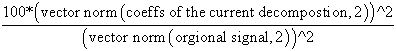3. Signal to noise ratio (SNR): This value gives the quality of the reconstructed signal. Higher the value, better. It is given by: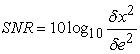Where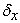and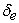are respectively the mean square of the speech signal and the mean square difference between the original and reconstructed signals.

4. Percentage of zero coefficient: it is given by the following relation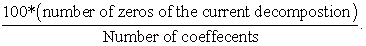### 3. Results and Discussion

Figure 2, Figure 3, Figure 4 and Figure 5 are shows a sample speech signal and approximations of the signal, at four different decomposition levels.

Choosing the right decomposition level in the DWT is important for many reasons. For processing audio signals no advantage is gained in going beyond scale 5 and usually processing at a lower scale leads to a better compression ratio

• Figure 2. MATLAB result at decompression level 1
• Figure 3. MATLAB result at decomposition level 2
• Figure 4. MATLAB result at decomposition level 3
• Figure 5. MATLAB result at decomposition level 5

The result shows the reconstructed approximation coefficients at different level for the sample speech signal analyzed and that the original speech data is still well represented by the level 1, 2, 3 and 5. When we compressed the original signal the approximation coefficient correctly represent in significant way at higher level.

3.1. Performance of Recorded Audio Coding

The performance of recorded audio coding for different Daubechies family along with RLE is shown in Table 2.

### 4. Conclusion

A suitable criterion used by for selecting optimal wavelets, is the energy retained in the first N/2 coefficients. Based on this criterion alone the daubechies 10 wavelet preserves perceptual information better than all the other wavelets tested.

The Db10 wavelet also provides the highest signal to noise ratio (SNR), peak signal to noise ratio (PSNR), and high percentage of zero coefficients. The Db10 wavelet has the highest no of vanishing moments of the wavelets tested and thus provides the most compact signal representation. Results show that in general there is improved in compression factor & signal to noise ratio of all daubechiles family (DB1, DB6, DB8, DB10) with DWT and RLE based technique.

### References

  J.I. Agbinya, “Discrete Wavelet Transform Techniques in Speech Processing”, IEEE Tencon Digital Signal Processing Applications Proceedings, IEEE, New York, NY, 1996, pp 514-519. In article  Othman O. Khalifa, SeringHabib Harding & Aisha Hassan A. Hashim “Compression using Wavelet Transform in Signal Processing’’: An International Journal, Volume (2): Issue (5). In article  D. Sinha and A. Tewfik. “Low Bit Rate Transparent Audio Compression using Adapted Wavelets”, IEEE Trans. ASSP, Vol. 41, No. 12, December 1993. In article View Article  Thyagaraian, K. S. “Still image and video compression with MATLAB” P.cm ISBN978-0-470-88692-2. In article  P. Srinivasan and L. H. Jamieson. “High Quality Audio Compression Using an Adaptive Wavelet Packet Decomposition and Psychoacoustic Modeling”, IEEE Transactions on Signal Processing, Vol 46, No. 4, April 1998. In article View Article  Martin Vetterli. “Still image and video compression with Matlab”, 2nd Edition. In article  HarmanpreetKaur and RamanpreetKaur, “Speech compression and decompression using DCT and DWT”, International Journal Computer Technology & Applicaton, Vol 3 (4), 1501-1503 IJCTA | July-August 2012. In article  Mark Nelson. “Data Compression” 1st Edition, ISBN 1558514341. In article  C.S. Burrus, R.A Gopinath, and H.Guo. “Introduction to Wavelet Transforms’’, Prentice Hall, Englewood Cliffs, New Jersey, 1998. In article  I. Daubechies. Ten Lectures on Wavelelet. SIAM, Philadelphia, PA, 1992. In article View Article  PubMedThis work is licensed under a Creative Commons Attribution 4.0 International License. To view a copy of this license, visit http://creativecommons.org/licenses/by/4.0/